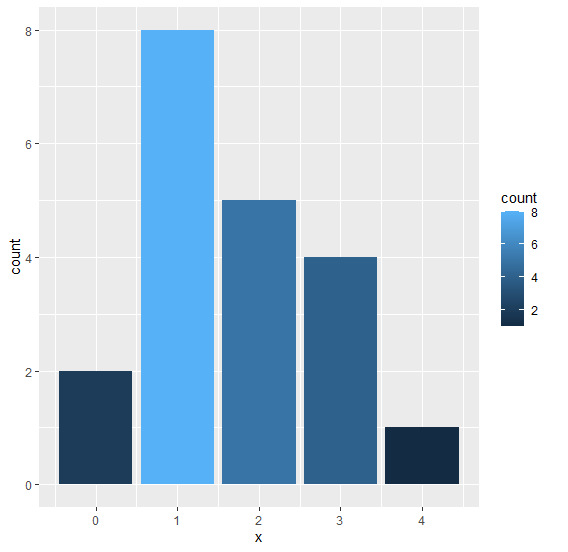# How to fill bars of a bar plot created using ggplot2 with colors based on frequency?

To fill bars in a bar plot using ggplot2 in R with colors based on frequency, we can use fill argument with count.

For Example, if we have a data frame called df that contains a single column X that contains repeated values and we want to create bar plot of values in X based on their frequencies then we can use the below command −

ggplot(df)+geom_bar(aes(X,fill=..count..))

## Example

Consider the data frame given below −

x<-rpois(20,2)
df<-data.frame(x)
df

The following dataframe is created

   x
1 1
2 2
3 2
4 3
5 1
6 0
7 3
8 3
9 3
10 2
11 1
12 1
13 1
14 2
15 1
16 1
17 0
18 2
19 4
20 1

To load the ggplot2 package and create bar chart filled with colors based on frequency of values in x on the above created data frame, add the following code to the above snippet −

x<-rpois(20,2)
df<-data.frame(x)
library(ggplot2)
ggplot(df)+geom_bar(aes(x,fill=..count..))

## Output

If you execute all the above given snippets as a single program, it generates the following Output −